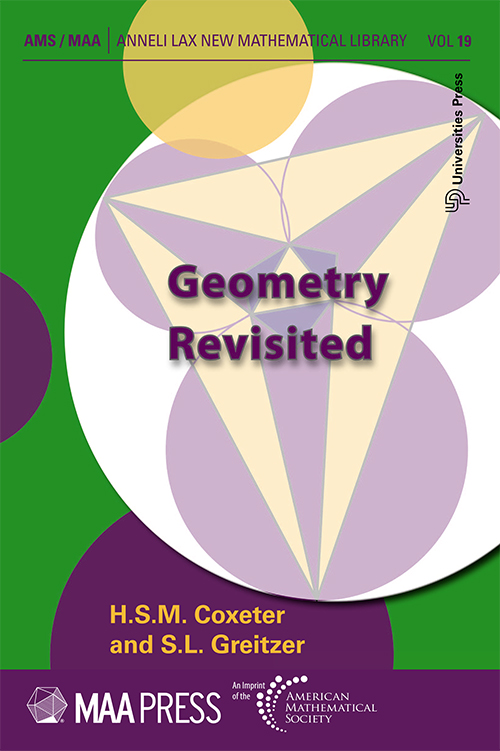## Geometry Revisited

#### Price: 700.00

Among the many beautiful and nontrivial theorems in geometry found in Geometry Revisited are the theorems of Ceva, Menelaus, Pappus, Desargues, Pascal, and Brianchon. A nice proof is given of Morleys remarkable theorem on angle trisectors. The transformational point of view is emphasized: reflections, rotations, translations, similarities, inversions, and affine and projective transformations. Many fascinating properties of circles, triangles, quadrilaterals, and conics are developed.

#### Contributors (Author(s), Editor(s), Translator(s), Illustrator(s) etc.)

H. S. M. Coxeter, S. L. Greitzer

Preface

Chapter 1 Points and Lines Connected with a Triangle

1.1 The extended Law of Sines
1.2 Ceva´s theorem
1.3 Points of interest
1.4 The incircle and excircles
1.5 The Steiner-Lehmus theorem
1.6 The orthic triangle
1.7 The medial triangle and Euler line
1.8 The nine-point circle
1.9 Pedal triangles

Chapter 2 Some Properties of Circles
2.1 The power of a point with respect to a circle
2.2 The radical axis of two circles
2.3 Coaxal circles
2.4 More on the altitudes and orthocenter of a triangle
2.5 Simson lines
2.6 Ptolemy´s theorem and its extension
2.7 More on Simson lines
2.8 The Butterfly
2.9 Morley´s theorem

Chapter 3 Collinearity and Concurrence
3.3 Napoleon triangles
3.4 Menelaus´s theorem
3.5 Pappus´s theorem
3.6 Perspective triangles; Desargues´s theorem
3.7 Hexagons
3.8 Pascal´s theorem
3.9 Brianchon´s theorem

Chapter 4 Transformations
4.1 Translation
4.2 Rotation
4.3 Half-tum
4.4 Reflection
4.5 Fagnano´s problem
4.6 The three jug problem
4.7 Dilatation
4.8 Spiral similarity
4.9 A genealogy of transformations

Chapter 5 An Introduction to Inversive Geometry
5.1 Separation
5.2 Cross ratio
5.3 Inversion
5.4 The inversive plane
5.5 Orthogonality
5.6 Feuerbach´s theorem
5.7 Coaxal circles
5.8 Inversive distance
5.9 Hyperbolic functions

Chapter 6 An Introduction to Projective Geometry
6.1 Reciprocation
6.2 The polar circle of a triangle
6.3 Conics 138 6.4 Focus and directrix
6.5 The projective plane
6.6 Central conics
6.7 Stereographic and gnomonic projection